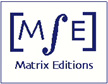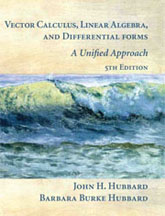Matrix Editions
serious mathematics,
written with the reader in mind

# Why a 5th edition?

### Vector Calculus, Linear Algebra, and Differential Forms: A Unified Approach, 5th editionJohn H. Hubbard and Barbara Burke Hubbard

ISBN 9780971576612

818 pages, Hardcover
8 x 10 inches, 2015, \$87

Return to the main page for this book

The initial impetus for producing a new edition rather than reprinting the fourth edition was to reconcile differences in numbering (pro­positions, examples, etc.) in the first and second printings of the fourth edition.

An additional impetus came from discussions John Hubbard had at an AMS meeting in Washington, DC, in October 2014. Mathematicians and computer scientists there told him that existing textbooks lack examples of "big matrices''. This led to two new examples illustrating the power of linear algebra and calculus: Example 2.7.12, showing how Google uses the Perron-Frobenius theorem to rank web pages, and Example 3.8.10, showing how the singular value decomposition (Theorem 3.8.1) can be used for computer face recognition.

The more we worked on the new edition, the more we wanted to change. An inclusive list is impossible; here are some additional highlights:

• In several places in the fourth edition (for instance, the proof of Proposition 6.4.8 on orientation-preserving parametrizations), we noted that "in Chapter 3 we failed to define the differentiability of functions defined on manifolds, and now we pay the price''. For this edition we "paid the price'' (Proposition and Definition 3.2.9) and the effort paid off handsomely, allowing us to shorten and simplify a number of proofs.

• We rewrote the discussion of multi-index notation in Section 3.3.

We rewrote the proof of Stokes's theorem and moved most of it out of the Appendix and into the main text.

We added Example 2.4.17 on Fourier series. We rewrote the discussion of classifying constrained critical points.

We use differentiation under the integral sign to compute the Fourier transform of the Gaussian, and discuss its relation to the Heisenberg uncertainty principle.

We added a new proposition (2.4.18) about orthonormal bases.

We greatly expanded the discussion of orthogonal matrices.

We added a new section in Chapter 3 on finite probability, showing the connection between probability and geometry. The new section also includes the statement and proof of the singular value decomposition.